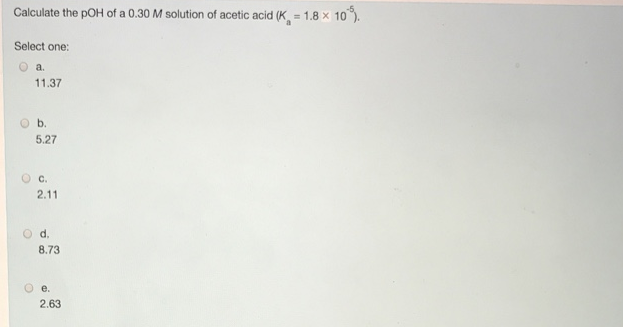# Problem: Calculate the pOH of a 0.30 M solution of acetic acid (Ka = 1.8 x 10-5). Select one: a. 11.37 b. 5.27 c. 2.11 d. 8.73 e. 2.63

###### FREE Expert Solution
89% (250 ratings)###### Problem Details

Calculate the pOH of a 0.30 M solution of acetic acid (Ka = 1.8 x 10-5). Select one:

a. 11.37

b. 5.27

c. 2.11

d. 8.73

e. 2.63